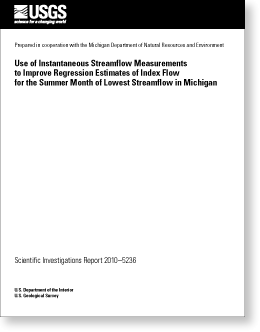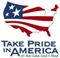Science Investigations Report 2010–5236

## Use of Instantaneous Streamflow Measurements to Improve Regression Estimates of Index Flow for the Summer Month of Lowest Streamflow in Michigan

### ABSTRACTIn Michigan, index flow Q50 is a streamflow characteristic defined as the minimum of median flows for July, August, and September. The state of Michigan uses index flow estimates to help regulate large (greater than 100,000 gallons per day) water withdrawals to prevent adverse effects on characteristic fish populations. At sites where long-term streamgages are located, index flows are computed directly from continuous streamflow records as GageQ50. In an earlier study, a multiple-regression equation was developed to estimate index flows IndxQ50 at ungaged sites. The index equation explains about 94 percent of the variability of index flows at 147 (index) streamgages by use of six explanatory variables describing soil type, aquifer transmissivity, land cover, and precipitation characteristics. This report extends the results of the previous study, by use of Monte Carlo simulations, to evaluate alternative flow estimators, DiscQ50, IntgQ50, SiteQ50, and AugmQ50. The Monte Carlo simulations treated each of the available index streamgages, in turn, as a miscellaneous site where streamflow conditions are described by one or more instantaneous measurements of flow. In the simulations, instantaneous flows were approximated by daily mean flows at the corresponding site. All estimators use information that can be obtained from instantaneous flow measurements and contemporaneous daily mean flow data from nearby long-term streamgages. The efficacy of these estimators was evaluated over a set of measurement intensities in which the number of simulated instantaneous flow measurements ranged from 1 to 100 at a site.

The discrete measurement estimator DiscQ50 is based on a simple linear regression developed between information on daily mean flows at five or more streamgages near the miscellaneous site and their corresponding GageQ50 index flows. The regression relation then was used to compute a DiscQ50 estimate at the miscellaneous site by use of the simulated instantaneous flow measurement. This process was repeated to develop a set of DiscQ50 estimates for all simulated instantaneous measurements, a weighted DiscQ50 estimate was formed from this set. Results indicated that the expected value of this weighted estimate was more precise than the IndxQ50 estimate for all measurement intensities evaluated.

The integrated index-flow estimator, IntgQ50, was formed by computing a weighted average of the index estimate IndxQ50 and the DiscQ50 estimate. Results indicated that the IntgQ50 estimator was more precise than the DiscQ50 estimator at low measurement intensities of one to two measurements. At greater measurement intensities, the precision of the IntgQ50 estimator converges to the DiscQ50 estimator. Neither the DiscQ50 nor the IntgQ50 estimators provided site-specific estimates. In particular, although expected values of DiscQ50 and IntgQ50 estimates converge with increasing measurement intensity, they do not necessarily converge to the site-specific value of Q50.

The site estimator of flow, SiteQ50, was developed to facilitate this convergence at higher measurement intensities. This is accomplished by use of the median of simulated instantaneous flow values for each measurement intensity level. A weighted estimate of the median and information associated with the IntgQ50 estimate was used to form the SiteQ50 estimate. Initial simulations indicate that the SiteQ50 estimator generally has greater precision than the IntgQ50 estimator at measurement intensities greater than 3, however, additional analysis is needed to identify streamflow conditions under which instantaneous measurements will produce estimates that generally converge to the index flows.

A preliminary augmented index regression equation was developed, which contains the index regression estimate and two additional variables associated with base-flow recession characteristics. When these recession variables were estimated as the medians of recession parameters computed from daily values defining recession events at index streamgages, this augmented estimator (AugIndxrY50), had 66.3-percent lower root mean square error (RMSE) than the IndxrY50 estimator. For the AugmrY50 estimator, in which the recession parameters for the augmented regression were estimated by Monte Carlo analysis, error characteristics decreased monotonically between an upper limit defined by error characteristics of the IndxrY50 and AugIndxrY50 estimators, depending on sampling intensity. Characterizing the uncertainty of new predicted values from the AugIndxrY50 estimator is problematic, however, because two of the key explanatory variables are uncertain.

Director, Michigan Water Science Center
U.S. Geological Survey
6520 Mercantile Way
Suite 5
Lansing, MI 48911-5991
http://mi.water.usgs.gov/

### Suggested citation:

Holtschlag, D.J., 2011, Use of instantaneous streamflow measurements to improve regression estimates of index flow for the summer month of lowest streamflow in Michigan: U.S. Geological Survey Scientific Investigations Report 2010–5236, 86 p.

### Contents

Abstract

Introduction

Flow Data

Improving Regression Estimates of Index Flow by use of Instantaneous Flow Measurements

Summary and Conclusions

References Cited

Appendix 1. Tables of streamgage attributes and flow characteristics

Appendix 2. Example Matlab Code computations of index flow measurements for improving regression estimates with simulated instantaneous measurements.U.S. Department of the Interior | U.S. Geological Survey
[an error occurred while processing this directive] URL: http://pubs.usgs.gov